Instructions

This problem set has three problems that you should submit an answer for (Spelling, Cancer and Jazz). Write your solutions to each of the three problems in separate documents and save them to files named p1.pdf, p2.pdf and p3.pdf.

Submission will work just like pset 1. See submitting for more details.

1. Earnest Spelling

The first functional spell checker was created in the early 1960s by Lester Earnest a professor in Stanford University's Artificial Intelligence lab. He wanted to create a system to recognize handwriting but realized that to do so, he also needed a spelling corrector! In this problem, we're going to look at how to model spelling correction as a Constraint Satisfaction Problem (CSP).Figure 1: Stanford AI lab where the first spell checker was installed.

You are given a sentence, which is a sequence of $n$ (possibly misspelled) words $w_1, \cdots, w_n$, where each $w_i$ is a sequence of letters. You have a dictionary $D$ of possible words. Define the edit distance $Edit(w,w')$ as the minimum number of insertions, deletions, or replacements required to convert $w$ into $w'$. Furthermore, you have a function $Fluency(w, w', w'')$, which measures how likely the words $w, w', w''$ are to appear next to each other in that order. Your goal is to find a sequence of real words from the dictionary $t_1 , \cdots , t_n$ that minimizes the sum of the edit distances $Edit(t_i, w_i)$ plus the sum of $-Fluency(t_i, t_{i+1}, t_{i+2})$. Note that this last term is the sum of the negative of the fluencies.
 a Formulate this problem as a weighted CSP. What are the variables, their domains, constraints and constraint weights? You should have $n$ variables, and no constraint should have arity of more than 3. b Suppose we add an additional requirement that at least one of the words of the spelling-corrected sentence must be a verb (let $V \subset D$ be the set of verbs). Add $O(n)$ variables and constraints with arity at most 3 to satisfy this requirement. c This problem could be modeled as a deterministic search problem (DSP) where the current state includes a cursor. The start state has the cursor on the first word. The set of legal actions only allows you to chose a word at the current cursor position. Briefly describe the pros and cons of modeling this problem using your formulation of a CSP versus the given DSP.

2. Cancer Warning Signs

One of the first uses of Bayesian networks was to model the transmission of genetic information from parent to child to better predict disease. In this problem, we will look at how inherited genetics affect breast cancer.

Every person has two "alleles" for each gene, one allele was passed from their mother and another from their father.

The presence of a damaged allele of the BRCA1 gene has been found to be associated with increased risk for breast cancer. Let $B$ represent a damaged allele in BRCA1, and $b$ represent a normal functional BRCA1 allele. Unless an individual has two functional alleles ($bb$), they will be particularly susceptible to cancer. Both men and women can develop breast cancer, but men have a much lower probability.

Let $G_x$ denote the two BRCA1 alleles for person $x$ (either $BB$, $Bb$, or $bb$). Let $C_x$ denote whether person $x$ will develop breast cancer (True or False). The Bayesian models below show three alternative representations of how the variables are related for two parents and one child.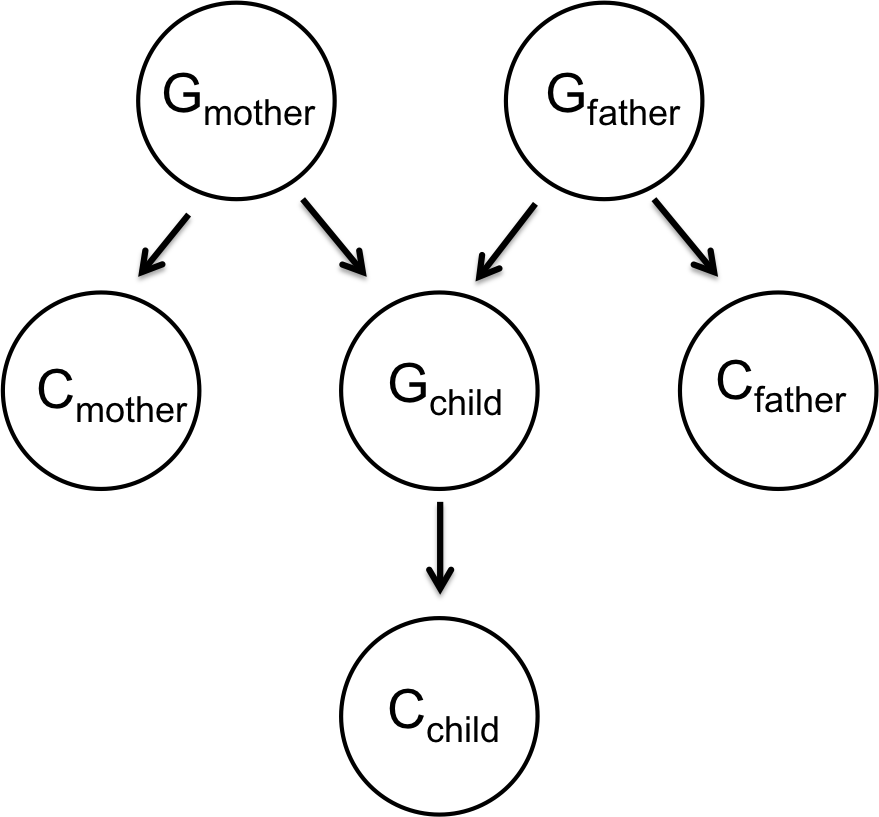modelA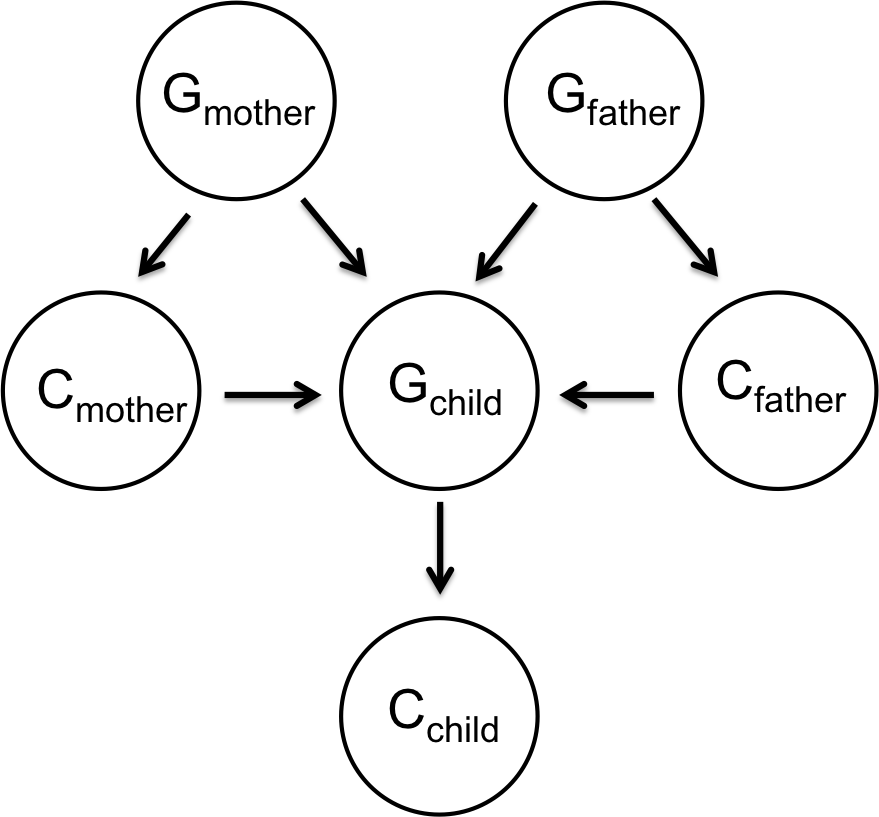modelB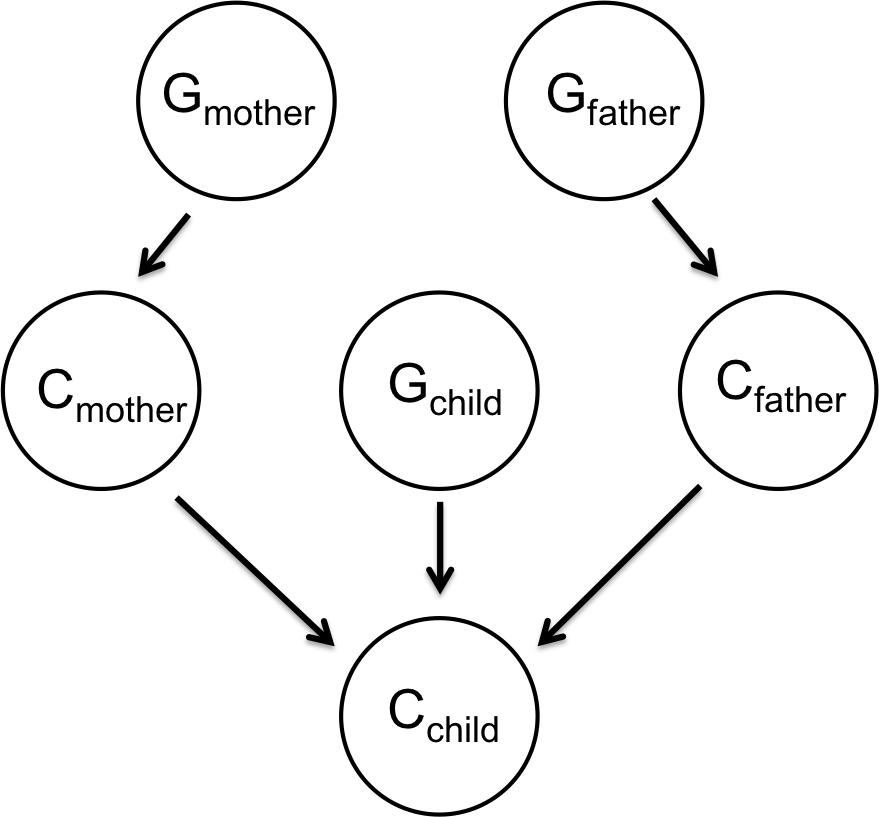modelC

Figure 2: Breast Cancer Models.

Parents transfer an allele to their child from their genotype with uniform probability, regardless of whether or not they have cancer. In other words, when a parent has a genotype $Bb$, then $B$ will be the allele they transfer to the child with probability 1/2 and $b$ will be the transferred allele with probability 1/2. When a parent has a genotype $BB$ or $bb$, the allele $B$ or $b$ respectively will be transferred with probability 1.

a Which of the provided networks claim $P(G_{mother}, G_{father}, G_{child}) = P(G_{mother})P(G_{father})P(G_{child})$?
b Which of the provided networks best represents how genetics and breast cancer are related?
c Suppose both parents are of genotype $Bb$. What is the conditional probability distribution (CPD) for the child's genotype?
d

We want to predict the chance of a male child developing breast cancer given that we have the following information: His father has breast cancer and his mother (who has been genetically tested) has two functional alleles $bb$. Assume that you know the emission probabilities of cancer given genome, and the prior probabilities for each allele combination:

Emission Probabilities Priors
$P(C_{father} = true \mid G_{father} = bb) = \theta_b$ $P(G_{father} = bb) = \phi_{bb}$
$P(C_{father} = true \mid G_{father} = Bb) = \theta_B$ $P(G_{father} = Bb) = \phi_{Bb}$
$P(C_{father} = true \mid G_{father} = BB) = \theta_B$ $P(G_{father} = BB) = \phi_{BB}$
The emission probabilities for the son are the same as for the father since they are both male.
 i Calculate the probabilities that the father has genotype $BB$, $Bb$, $bb$, given that we know he has breast cancer. ii Calculate the probabilities that the child has genotype $BB$, $Bb$, $bb$, given that we know the mother is $bb$, and that the father has the genotype distribution from part (i). iii What is the probability that the son will develop breast cancer given that his father has breast cancer and his mother is $bb$?

3. Jazz Improvisation

Jazz is a celebration of the eclectic, and as a genre it is relatively free of typical compositional rules. As such it is a domain where computers are able to make creative contributions.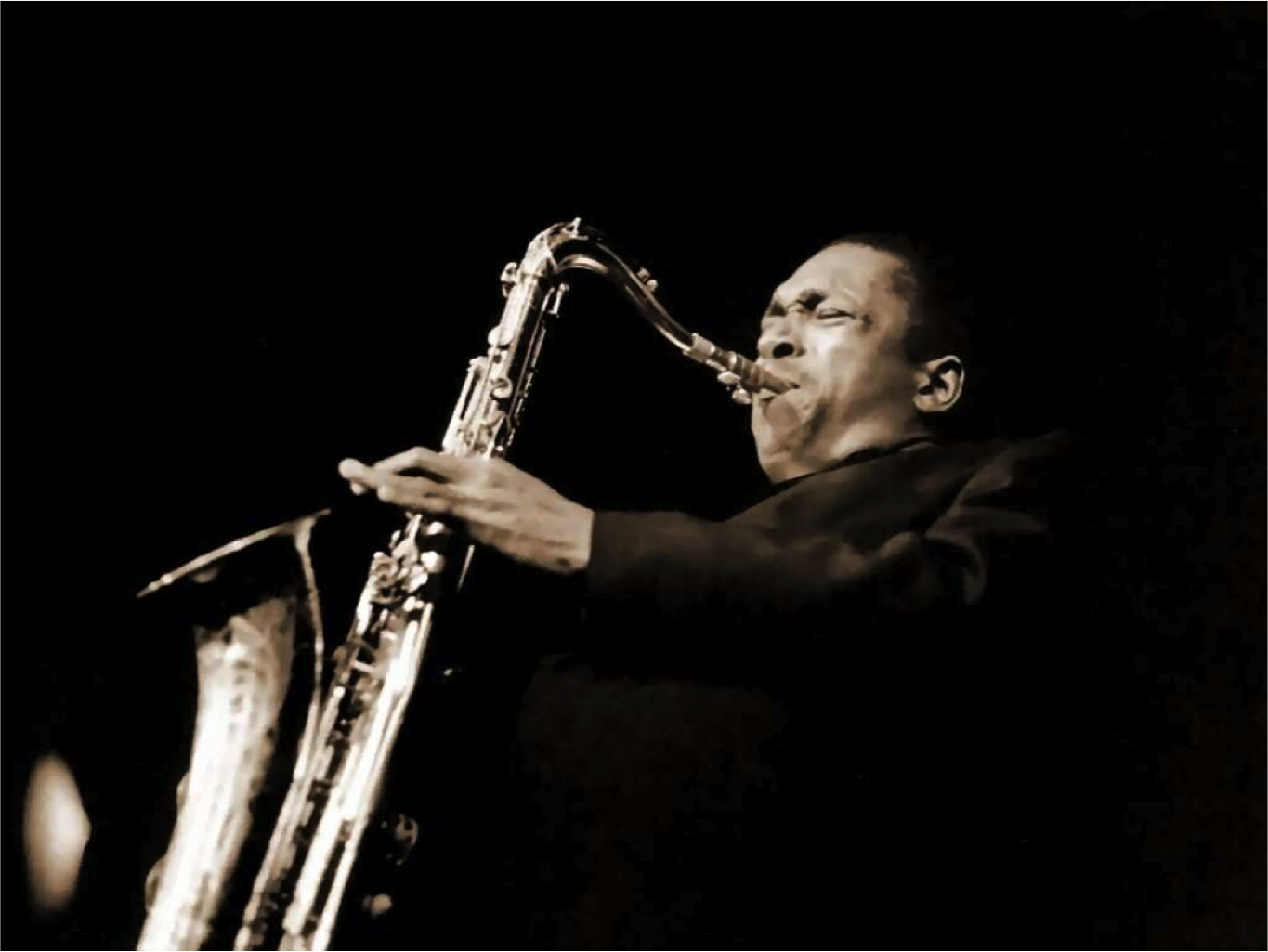Figure 3: John Coltrane on Sax.

In this problem, you are going to write a problem that can improvize jazz music for a saxophone using Markov chains. Assume you have a dictionary of notes $D$ = {C, E, E♭, G, G♯, B, rest} (this augmented scale is prevalent in John Coltrane songs). For simplicity we are going to assume each note is a quarter note.

 a In our first model each note is generated by a probability distribution that is dependent only on the previous note. The first note in the improvisation is generated randomly from the dictionary of notes. Here is the Markov Chain model: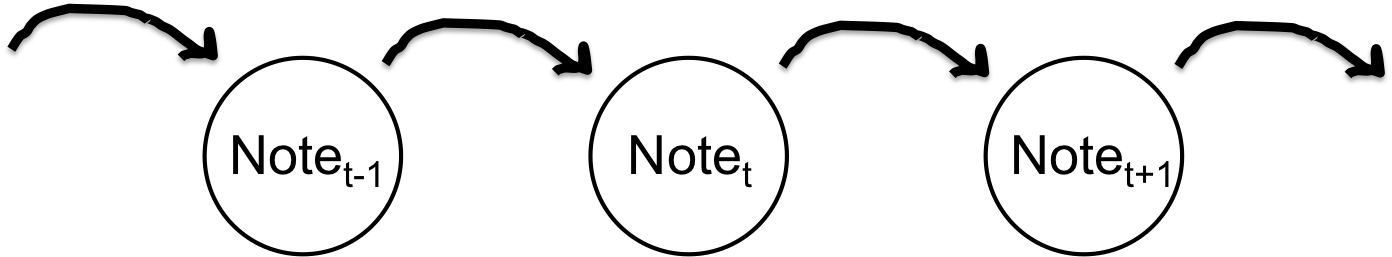Figure 4: Simple Jazz Markov Network. Describe the Conditional Probability Table for all nodes in the network. Explain how you could use this model to generate a jazz solo with $N$ notes. b Generating a note based only on the last note creates choppy jazz. To fix this, we want to change our model to generate a new note based on the last three notes played (where the order of those three notes matters). Formalize this new model: describe your variables and for each variable describe its CPT. Draw a directed graph which visualizes your model. c If you have access to the notes played in hundreds of hours of songs (of the same jazz style). How would you learn the transition probability for your model in part b? d Challenging: In order to improve our composition, we need to first learn how to listen. We are given a score $S = [n_1, n_2 \cdots n_L]$ of notes $n_i$ that a musician plans to play. At any time, the musician may play the current note in the score, add in an extra note or skip the current note. Let $M = [m_1, m_2, \cdots, m_T]$ be the observed notes $m_i$ the musician actually plays. Write a hidden Markov model (describe variables and CPTs) that will allow you to track the hidden note in the score the musician is on given the observed notes. e We chose to track where in a score a musician is using a particle filter. Describe the structure of a particle, initialization, update and transition steps.